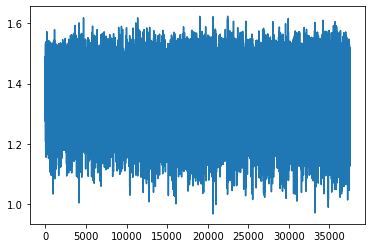# Some how my model isn't updating

Hello. I am training a NN classifier and everything seems to work fine. Except that my model isn’t updating(the losses aren’t dropping from the beginning).

``````class NN(nn.Module):
def __init__(self,width = 128):
super().__init__()

self.fc1 = nn.Sequential(
nn.Linear(50,512),
nn.ReLU(),
nn.Linear(512,512),
nn.ReLU(),
nn.Linear(512,512),
nn.ReLU(),
nn.Linear(512,256),
nn.ReLU(),
nn.Linear(256,256),
nn.ReLU(),
nn.Linear(256,128),
nn.ReLU(),
nn.Linear(128,64),
nn.ReLU(),
nn.Linear(64,32),
nn.ReLU(),
nn.Linear(32,16),
nn.ReLU(),
nn.Linear(16,4),
nn.Softmax(dim = 1)
)

def forward(self, x):

out = self.fc1(x)

return out

``````
``````net = NN().to(device)
loss_function = nn.CrossEntropyLoss(weight=weight)
``````
``````plot_loss = []
for epoch in range(3): # 3 full passes over the data

for data in trainset:  # `data` is a batch of data
X, y = data  # X is the batch of features, y is the batch of targets.

X = X.to(device)
y = y.to(device)

net.zero_grad()  # sets gradients to 0 before loss calc. You will do this likely every step.
output = net(X.view(-1,50))  # pass in the reshaped batch (recall they are 28x28 atm)

loss = loss_function(output, y.flatten())  # calc and grab the loss value

loss.backward()  # apply this loss backwards thru the network's parameters
optimizer.step()  # attempt to optimize weights to account for loss/gradients
plot_loss.append(loss)
print(loss)  # print loss. We hope loss (a measure of wrong-ness) declines!
``````

the loss after 3 epoch:Thanks in advance for any help.

What do you classify?

I am trying to classify features into four categories(0~3). This is the sample data. (a batch)